# RD Sharma Solutions for Class 8 Maths Chapter 12 Percentage

In the previous class, we have studied the concept of percentage. We have also learnt to convert a percent into a fraction, a ratio or decimal and vice-versa. Now in this chapter, we shall discuss more problems based on percentage. Students who struggle to solve problems have arrived at the right platform. The solutions here are solved and prepared by our expert tutors at BYJU’S in a step by step format to help students understand the concepts clearly. When it comes to preparing for the exam, students can refer to RD Sharma Class 8, which is the best study material prepared by our experts, in accordance with the latest CBSE patterns. The PDFs of this chapter are available here, where students can download for free from the links given below.

Chapter 12- Percentage contains two exercises and the RD Sharma Solutions present in this page provide solutions to the questions present in each exercise. Now, let us have a look at the concepts discussed in this chapter.

• Express percent as a fraction.
• Express percent as a ratio.
• Express percent in decimal form.
• Finding a percentage of a number.

## Download the pdf of RD Sharma Solutions For Class 8 Maths Chapter 12 Percentage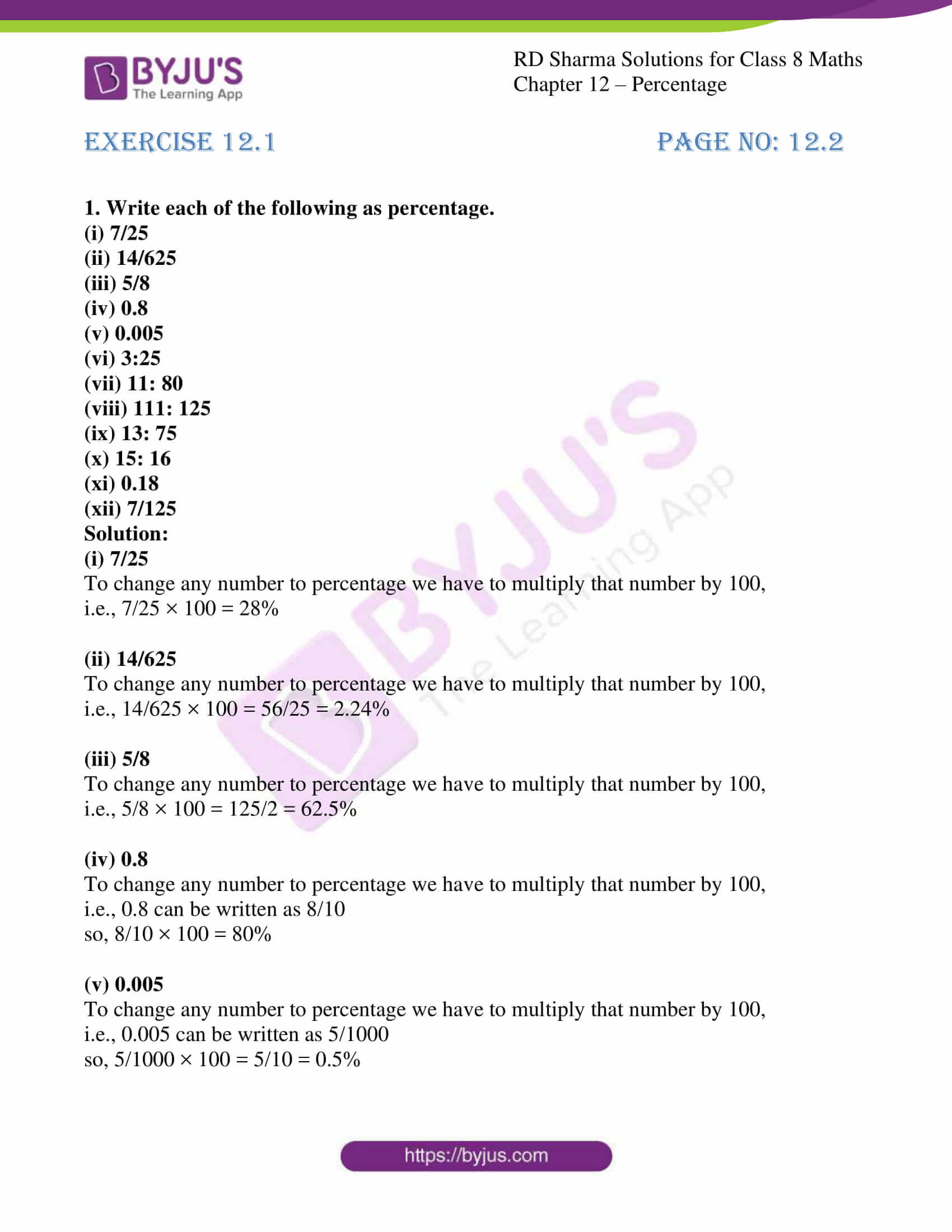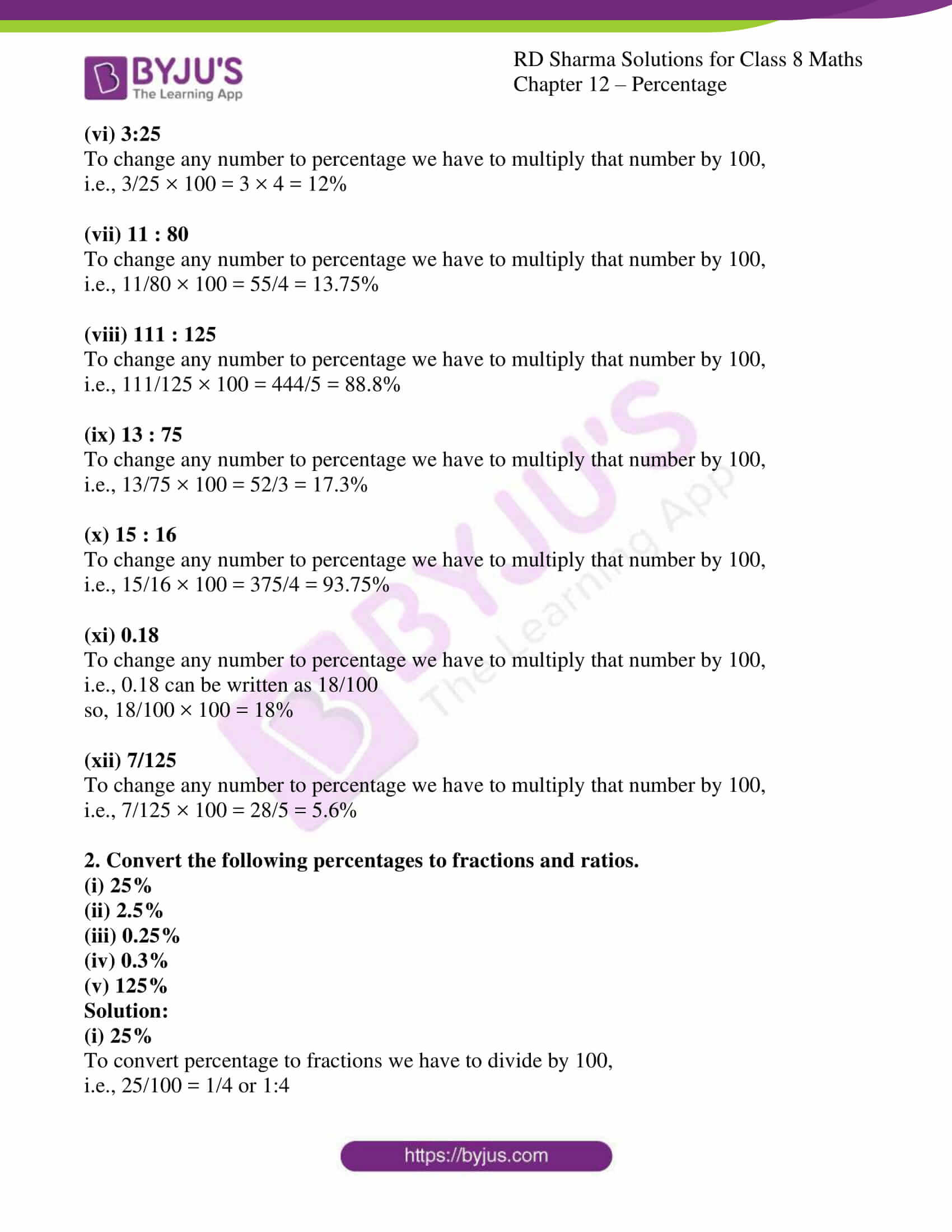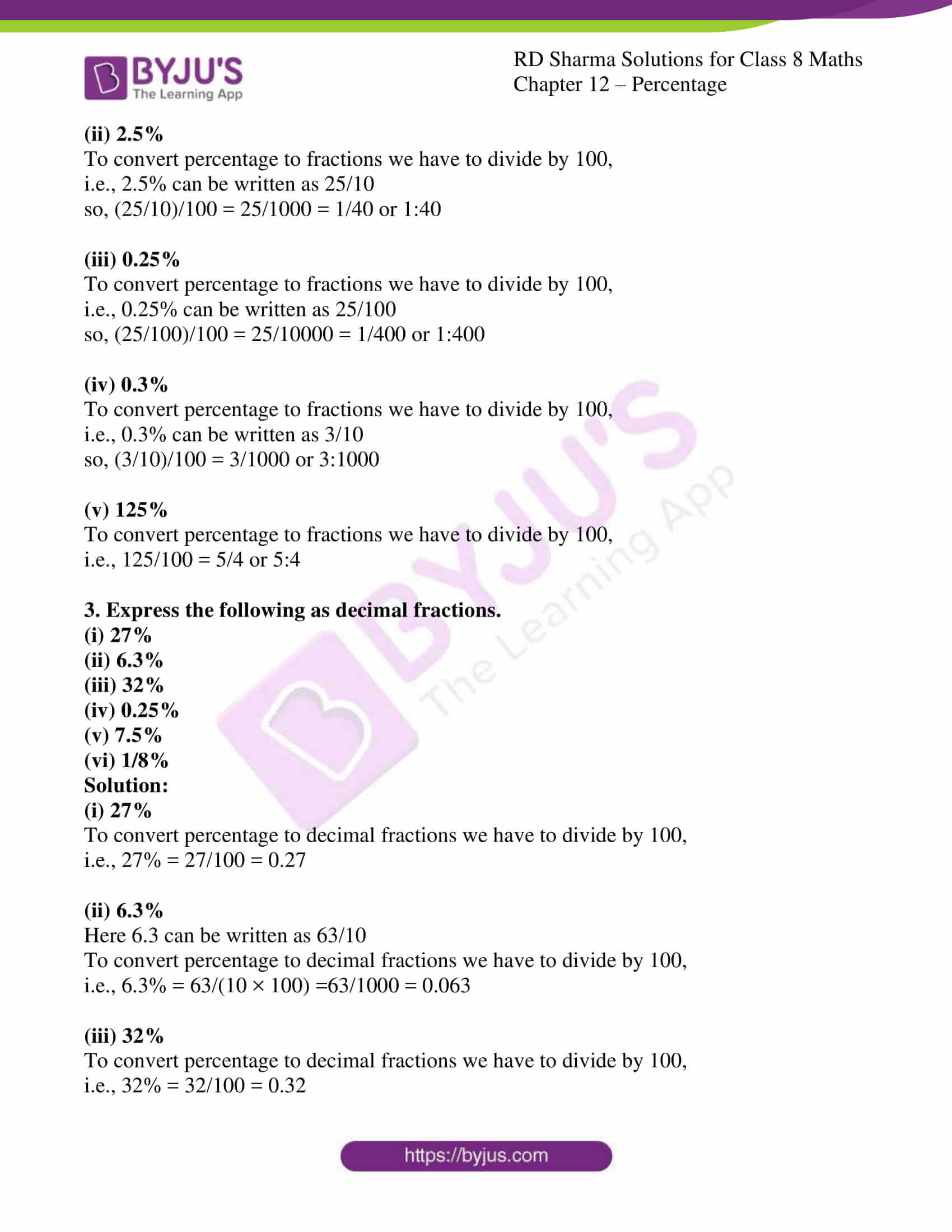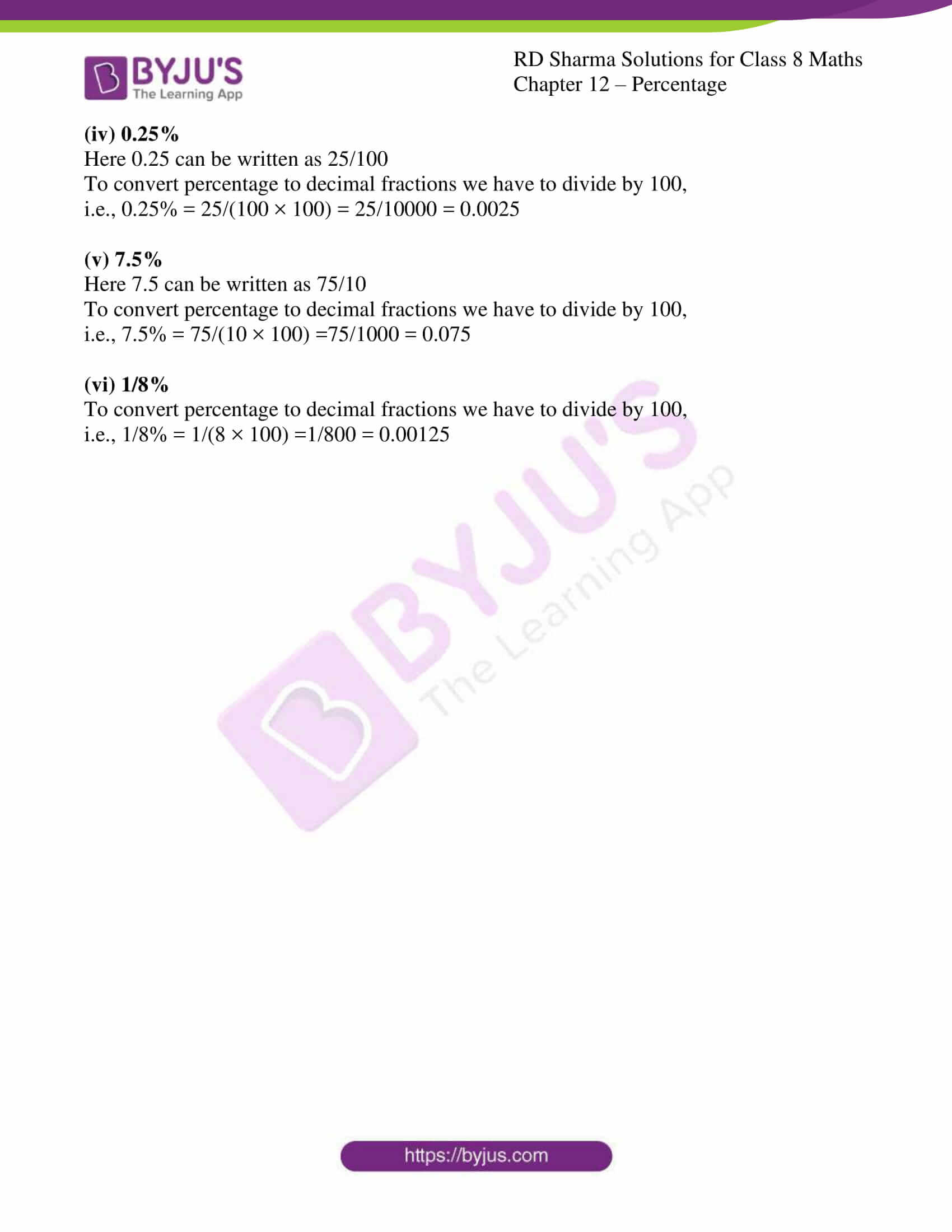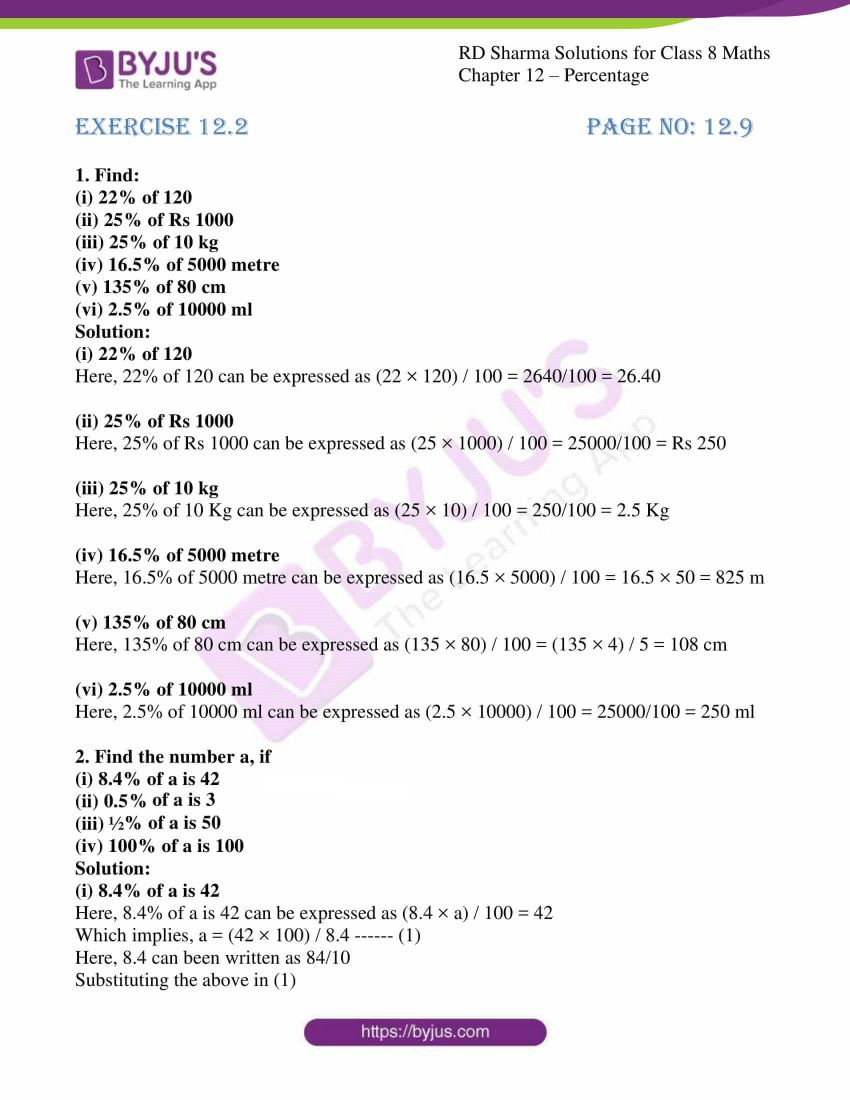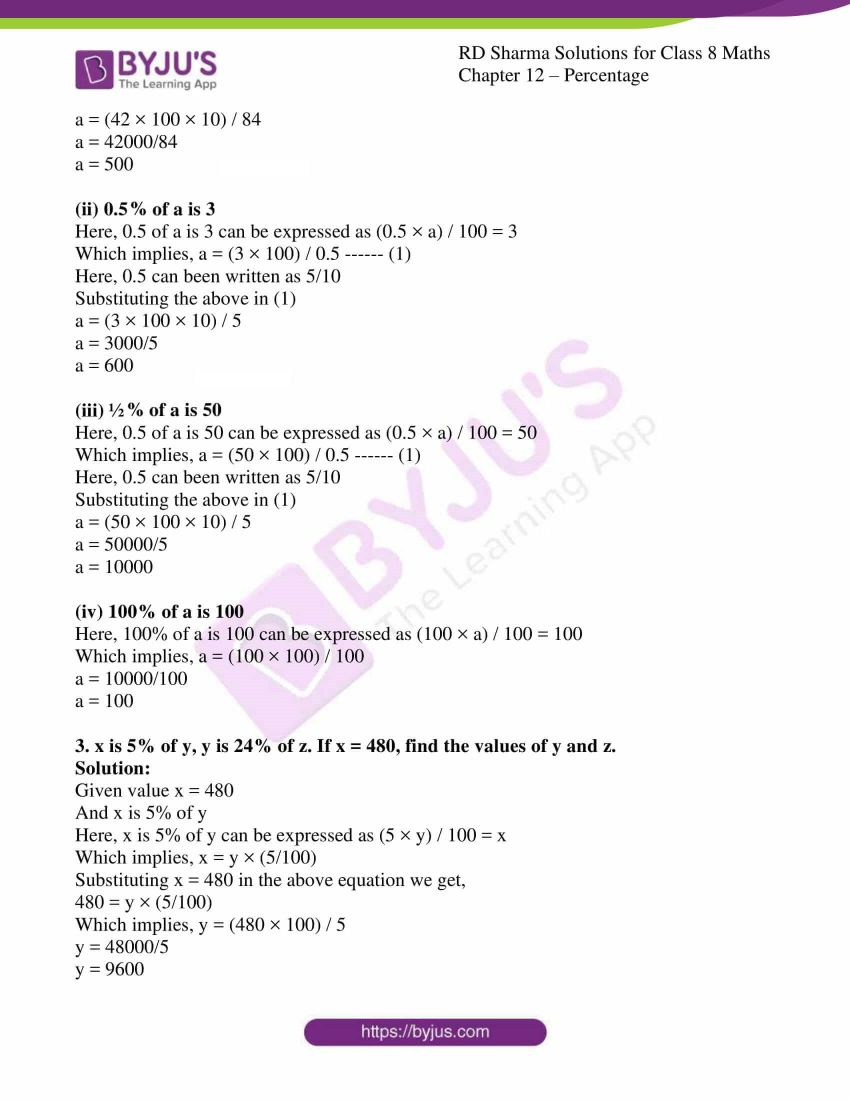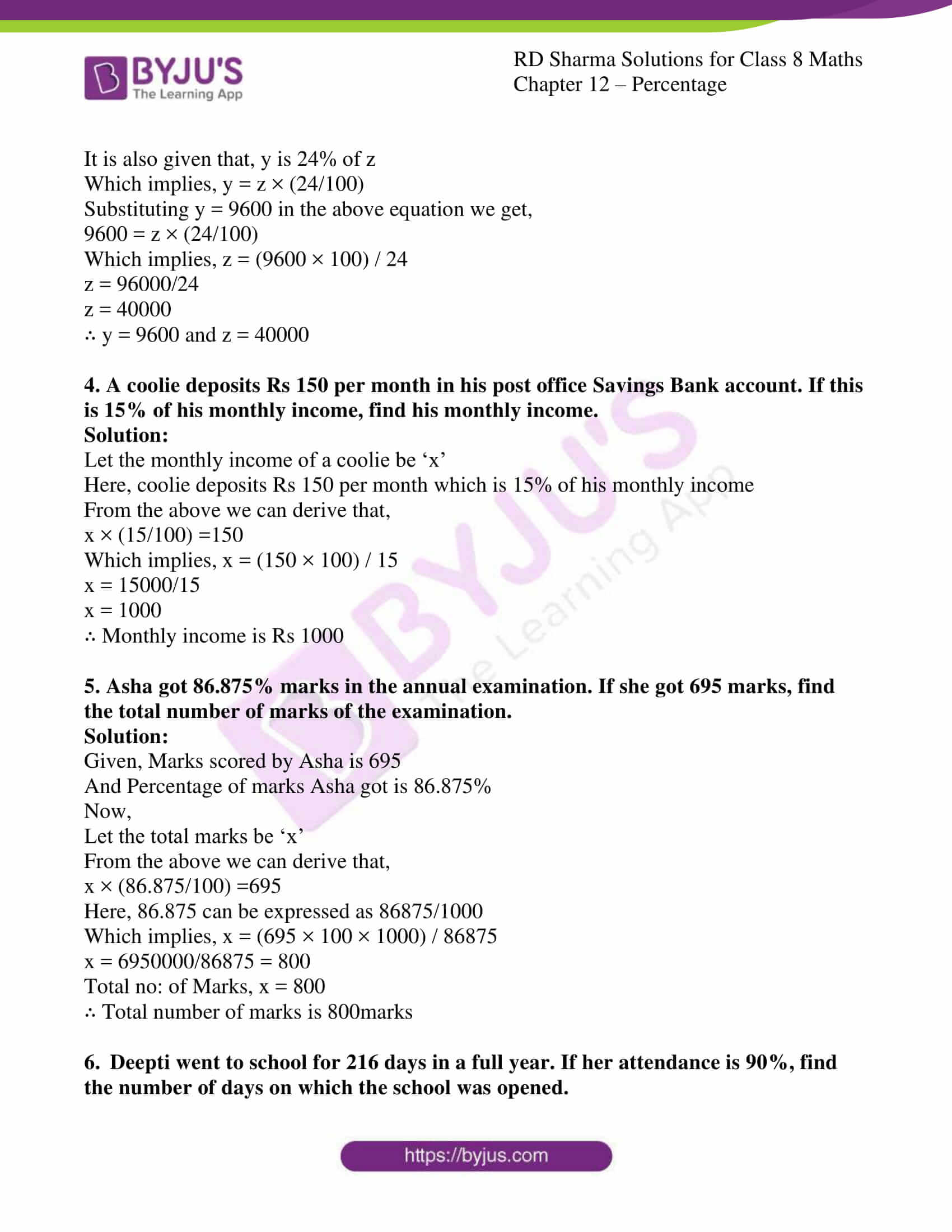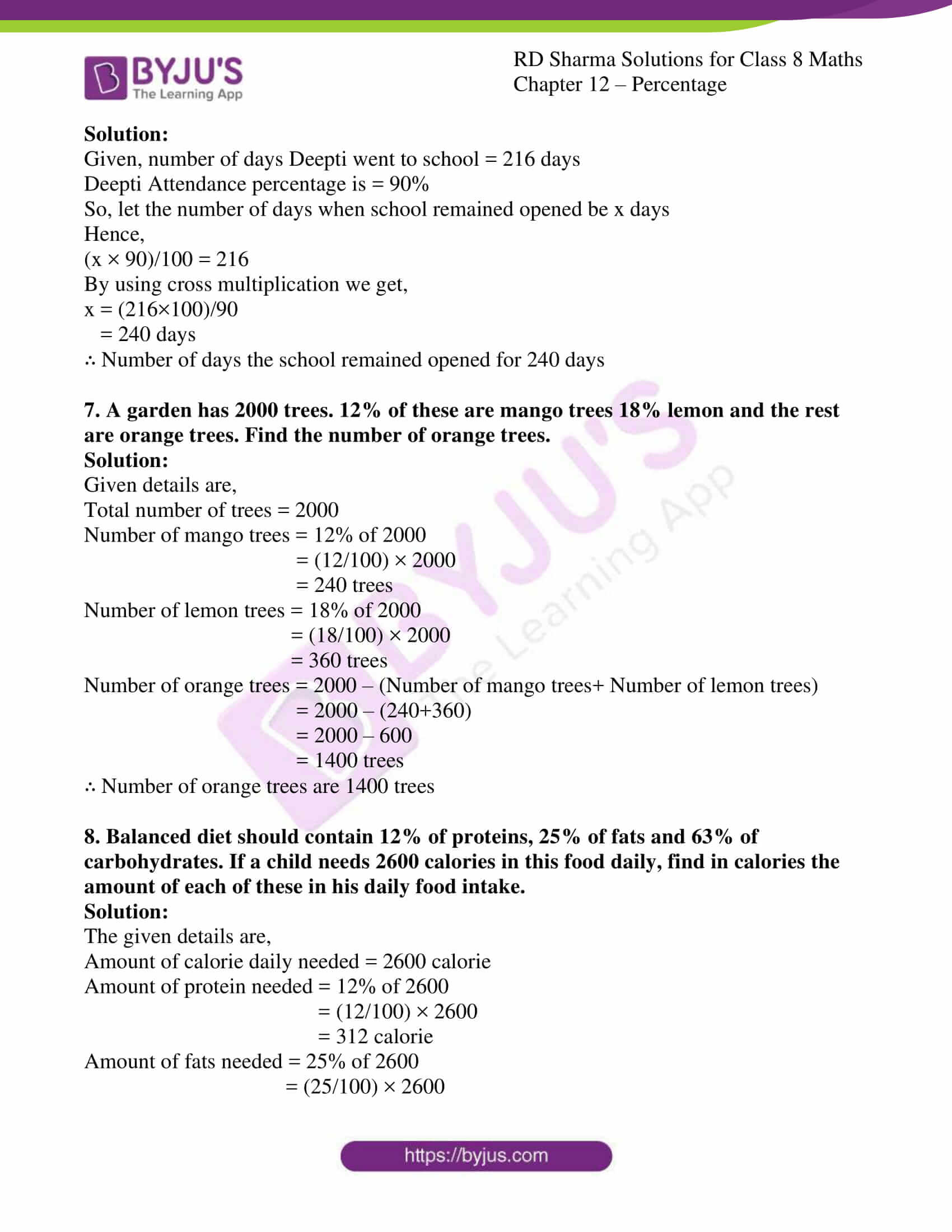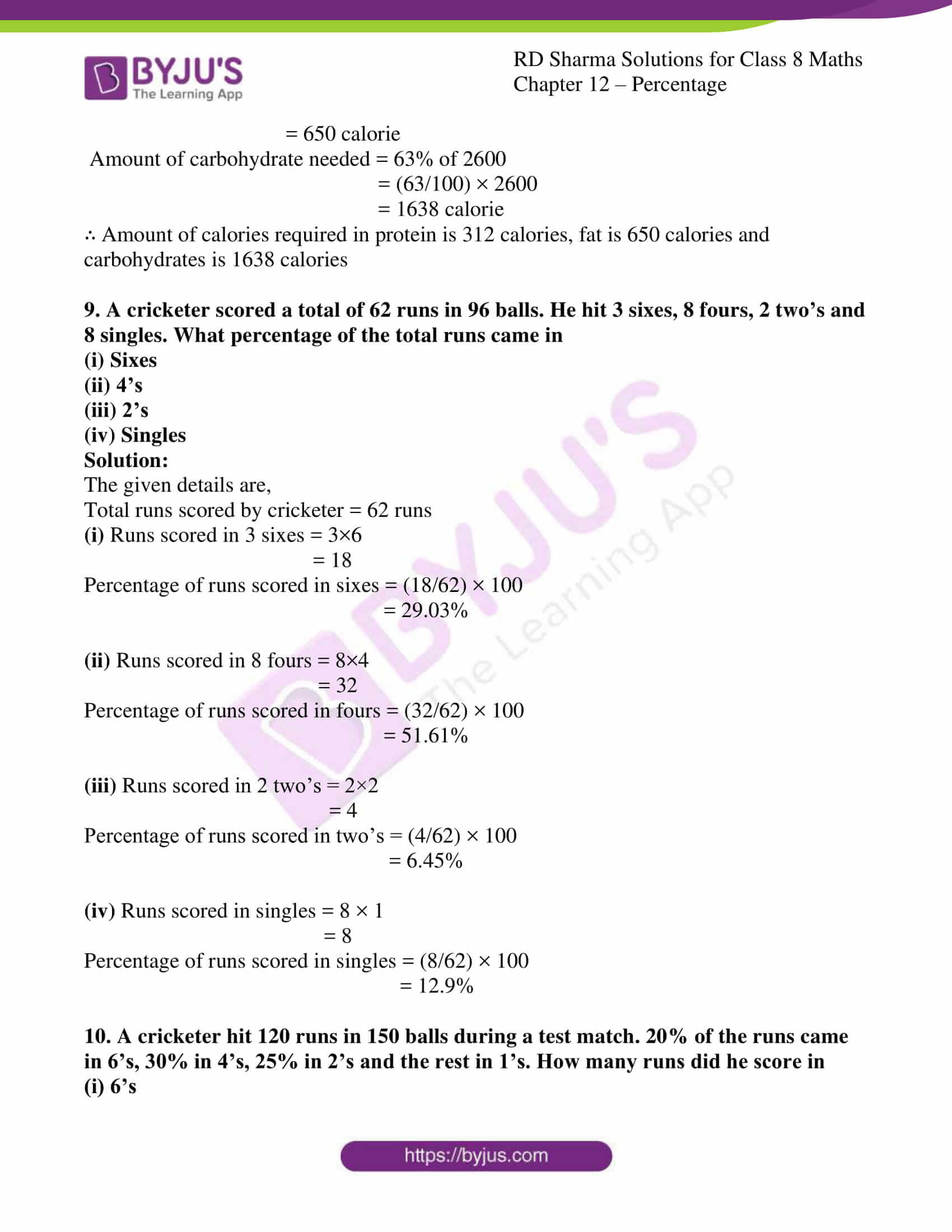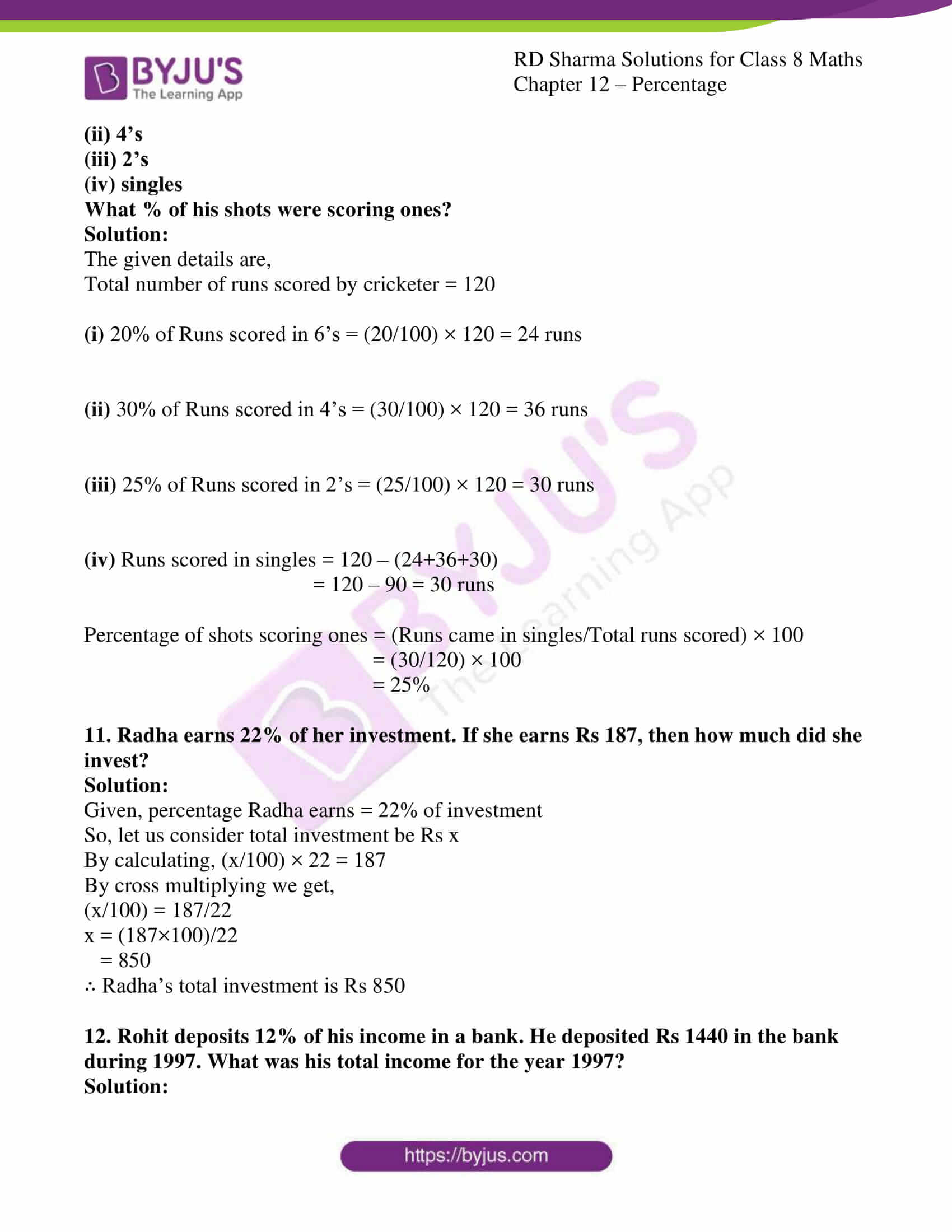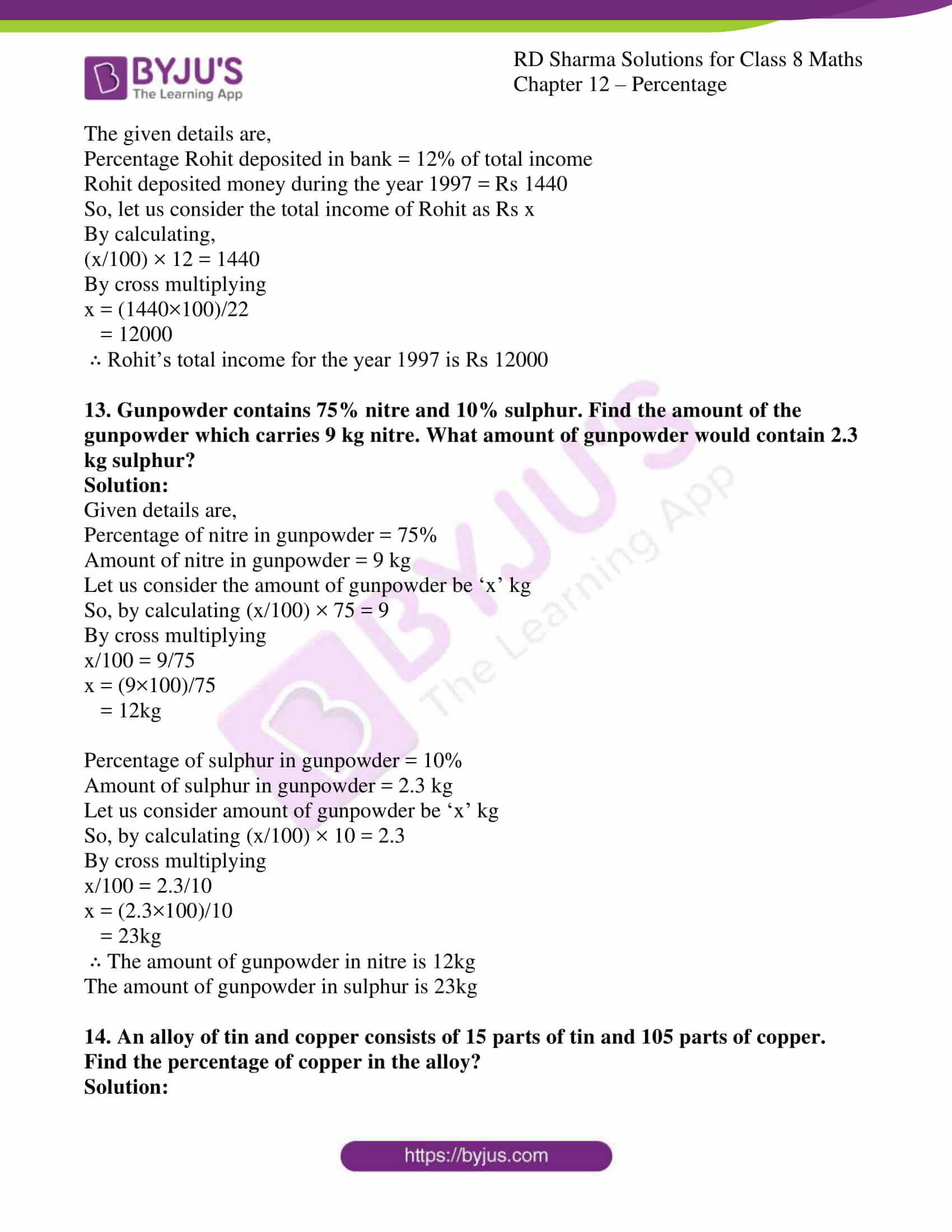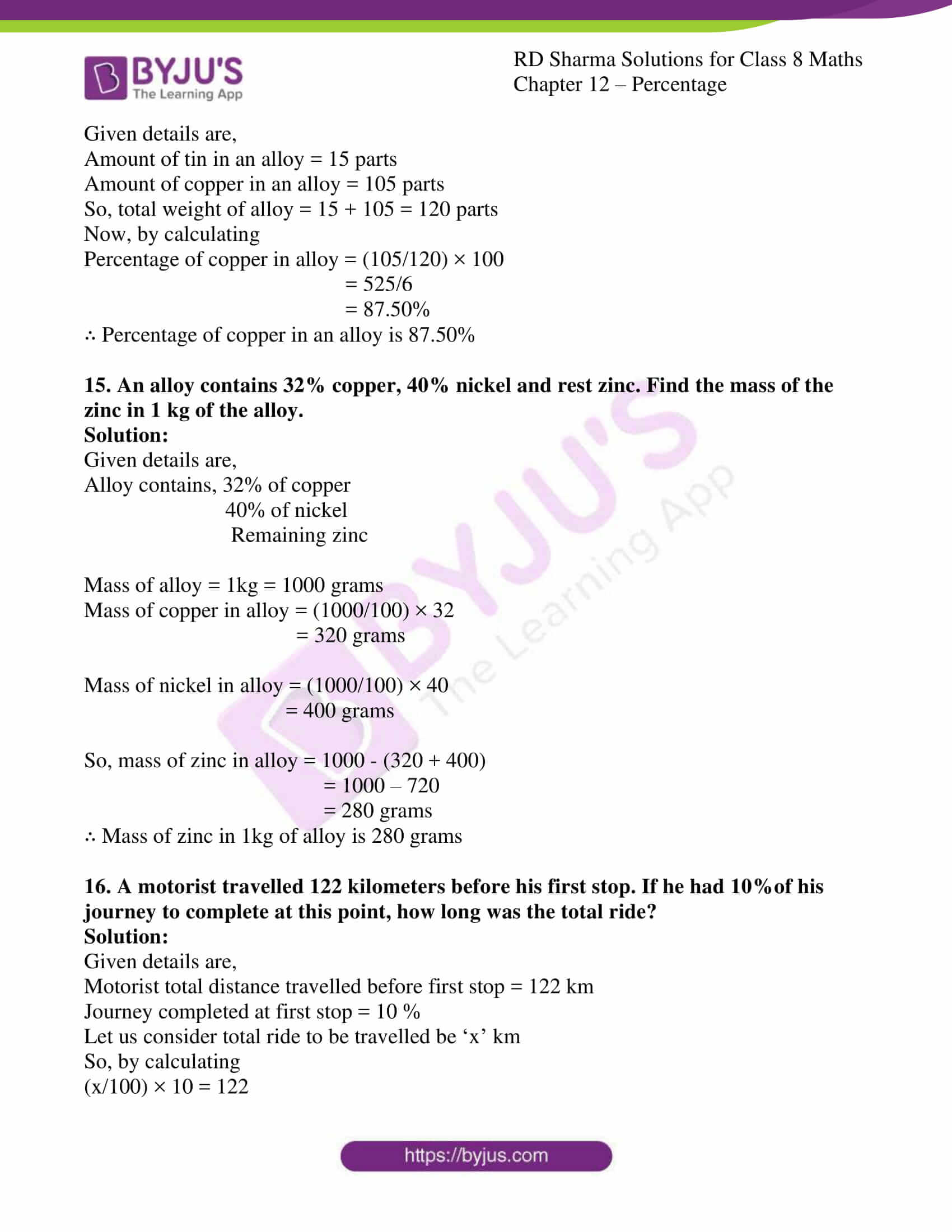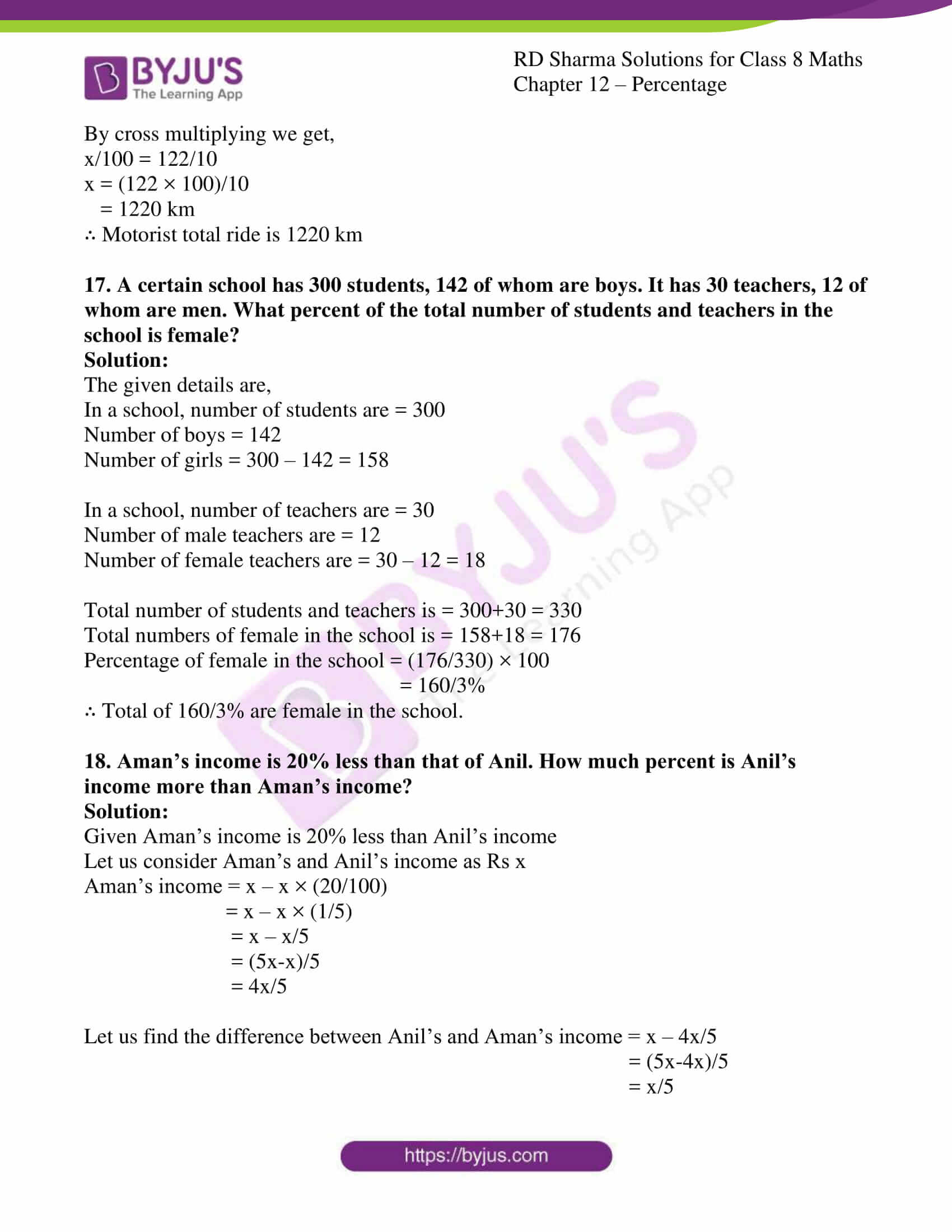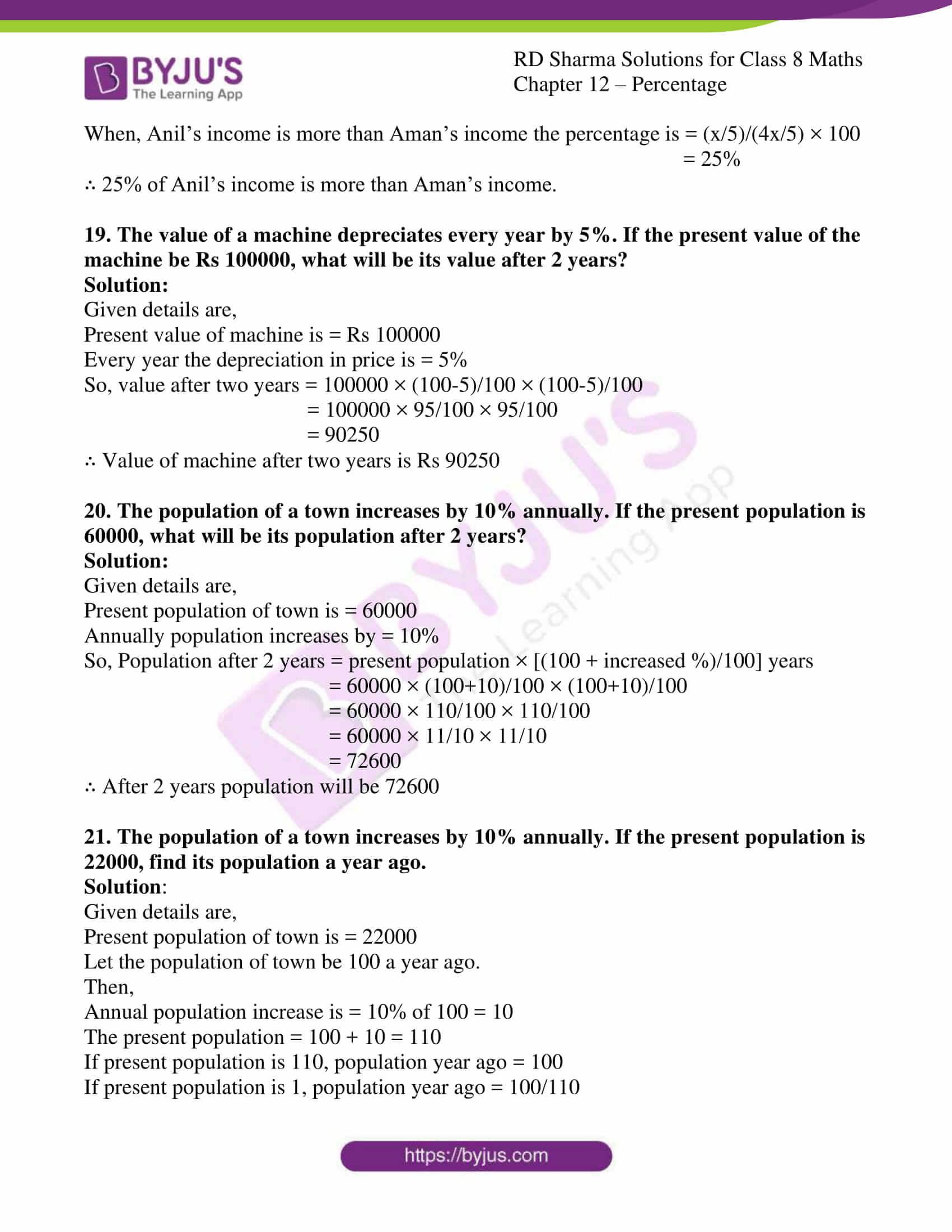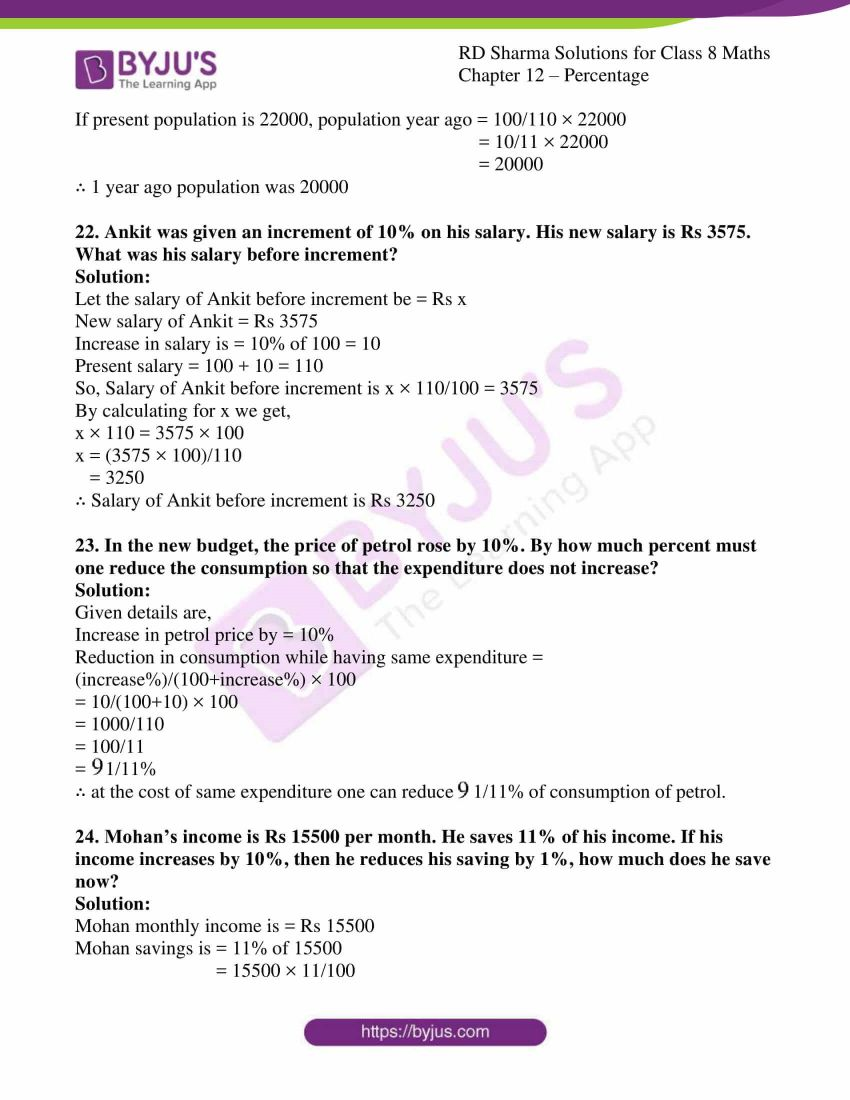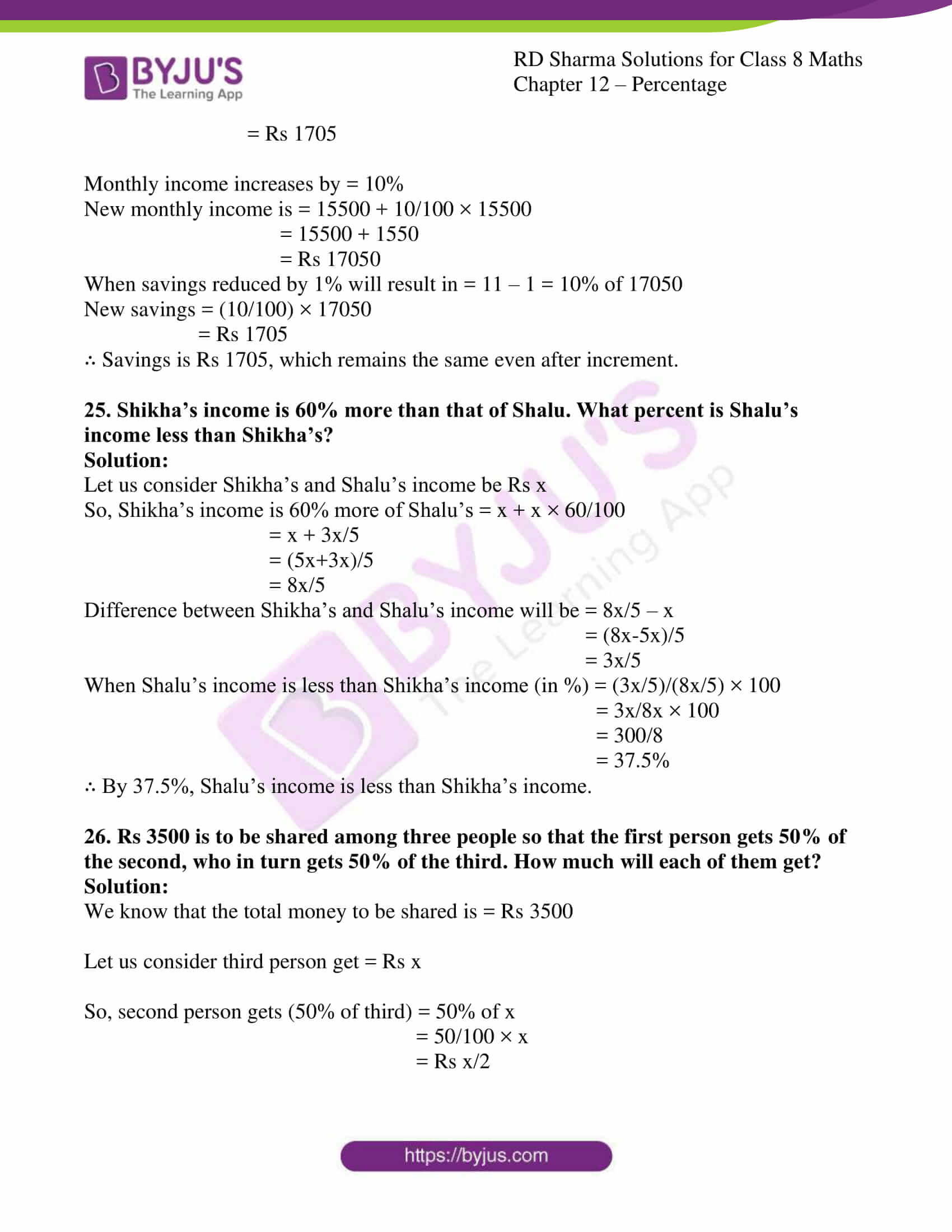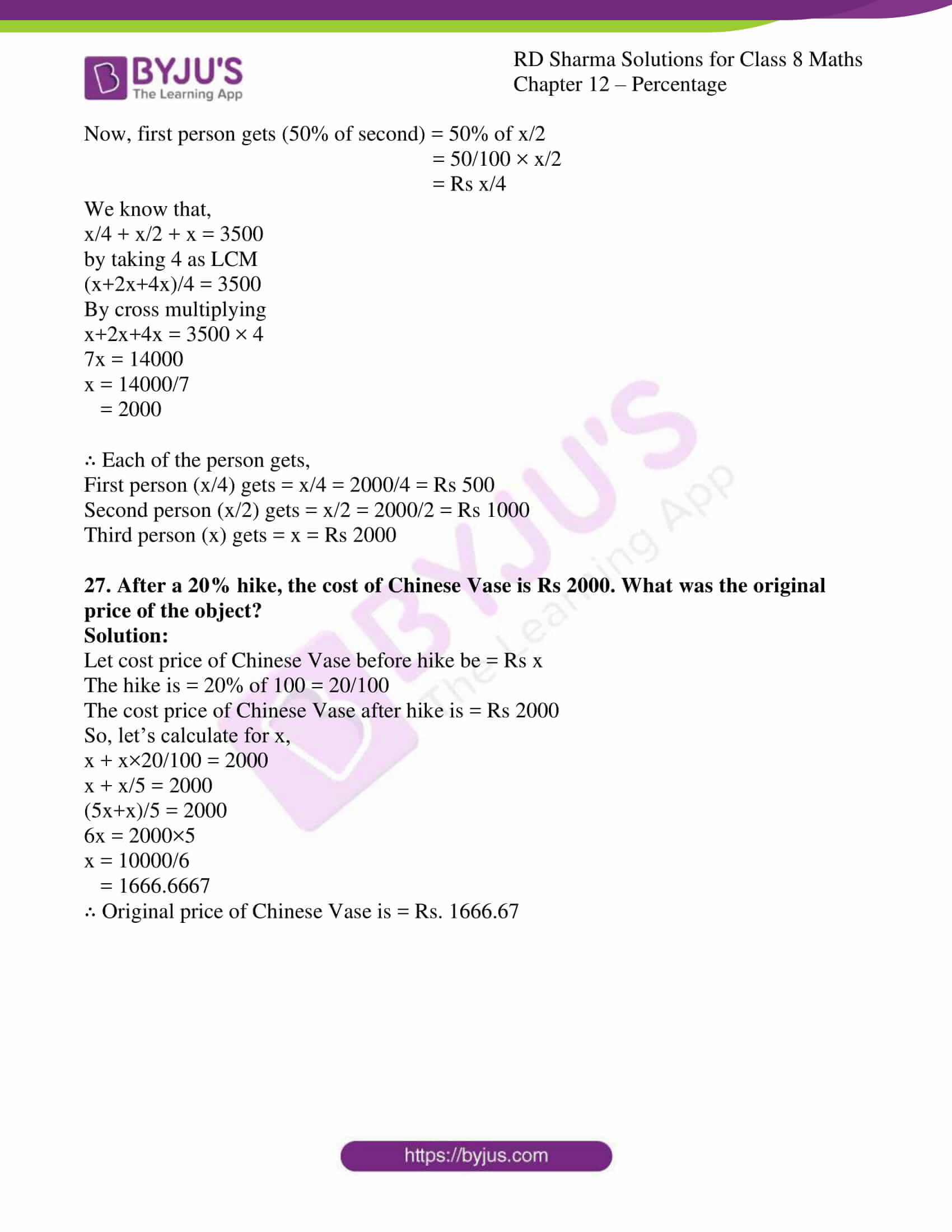### Access answers to Maths RD Sharma Solutions For Class 8 Chapter 12 Percentage

EXERCISE 12.1 PAGE NO: 12.2

1. Write each of the following as percentage.
(i) 7/25
(ii) 14/625
(iii) 5/8
(iv) 0.8
(v) 0.005
(vi) 3:25
(vii) 11: 80
(viii) 111: 125
(ix) 13: 75
(x) 15: 16
(xi) 0.18
(xii) 7/125

Solution:

(i) 7/25

To change any number to percentage we have to multiply that number by 100,

i.e., 7/25 × 100 = 28%

(ii) 14/625

To change any number to percentage we have to multiply that number by 100,

i.e., 14/625 × 100 = 56/25 = 2.24%

(iii) 5/8

To change any number to percentage we have to multiply that number by 100,

i.e., 5/8 × 100 = 125/2 = 62.5%

(iv) 0.8

To change any number to percentage we have to multiply that number by 100,

i.e., 0.8 can be written as 8/10

so, 8/10 × 100 = 80%

(v) 0.005

To change any number to percentage we have to multiply that number by 100,

i.e., 0.005 can be written as 5/1000

so, 5/1000 × 100 = 5/10 = 0.5%

(vi) 3:25

To change any number to percentage we have to multiply that number by 100,

i.e., 3/25 × 100 = 3 × 4 = 12%

(vii) 11 : 80

To change any number to percentage we have to multiply that number by 100,

i.e., 11/80 × 100 = 55/4 = 13.75%

(viii) 111 : 125

To change any number to percentage we have to multiply that number by 100,

i.e., 111/125 × 100 = 444/5 = 88.8%

(ix) 13 : 75

To change any number to percentage we have to multiply that number by 100,

i.e., 13/75 × 100 = 52/3 = 17.3%

(x) 15 : 16

To change any number to percentage we have to multiply that number by 100,

i.e., 15/16 × 100 = 375/4 = 93.75%

(xi) 0.18

To change any number to percentage we have to multiply that number by 100,

i.e., 0.18 can be written as 18/100

so, 18/100 × 100 = 18%

(xii) 7/125

To change any number to percentage we have to multiply that number by 100,

i.e., 7/125 × 100 = 28/5 = 5.6%

2. Convert the following percentages to fractions and ratios.
(i) 25%
(ii) 2.5%
(iii) 0.25%
(iv) 0.3%
(v) 125%

Solution:

(i) 25%

To convert percentage to fractions we have to divide by 100,

i.e., 25/100 = 1/4 or 1:4

(ii) 2.5%

To convert percentage to fractions we have to divide by 100,

i.e., 2.5% can be written as 25/10

so, (25/10)/100 = 25/1000 = 1/40 or 1:40

(iii) 0.25%

To convert percentage to fractions we have to divide by 100,

i.e., 0.25% can be written as 25/100

so, (25/100)/100 = 25/10000 = 1/400 or 1:400

(iv) 0.3%

To convert percentage to fractions we have to divide by 100,

i.e., 0.3% can be written as 3/10

so, (3/10)/100 = 3/1000 or 3:1000

(v) 125%

To convert percentage to fractions we have to divide by 100,

i.e., 125/100 = 5/4 or 5:4

3. Express the following as decimal fractions.
(i) 27%
(ii) 6.3%
(iii) 32%

(iv) 0.25%
(v) 7.5%
(vi) 1/8%

Solution:

(i) 27%

To convert percentage to decimal fractions we have to divide by 100,

i.e., 27% = 27/100 = 0.27

(ii) 6.3%

Here 6.3 can be written as 63/10

To convert percentage to decimal fractions we have to divide by 100,

i.e., 6.3% = 63/(10 × 100) =63/1000 = 0.063

(iii) 32%

To convert percentage to decimal fractions we have to divide by 100,

i.e., 32% = 32/100 = 0.32

(iv) 0.25%

Here 0.25 can be written as 25/100

To convert percentage to decimal fractions we have to divide by 100,

i.e., 0.25% = 25/(100 × 100) = 25/10000 = 0.0025

(v) 7.5%

Here 7.5 can be written as 75/10

To convert percentage to decimal fractions we have to divide by 100,

i.e., 7.5% = 75/(10 × 100) =75/1000 = 0.075

(vi) 1/8%

To convert percentage to decimal fractions we have to divide by 100,

i.e., 1/8% = 1/(8 × 100) =1/800 = 0.00125

EXERCISE 12.2 PAGE NO: 12.9

1. Find:
(i) 22% of 120
(ii) 25% of Rs 1000
(iii) 25% of 10 kg
(iv) 16.5% of 5000 metre
(v) 135% of 80 cm
(vi) 2.5% of 10000 ml

Solution:

(i) 22% of 120

Here, 22% of 120 can be expressed as (22 × 120) / 100 = 2640/100 = 26.40

(ii) 25% of Rs 1000

Here, 25% of Rs 1000 can be expressed as (25 × 1000) / 100 = 25000/100 = Rs 250

(iii) 25% of 10 kg

Here, 25% of 10 Kg can be expressed as (25 × 10) / 100 = 250/100 = 2.5 Kg

(iv) 16.5% of 5000 metre

Here, 16.5% of 5000 metre can be expressed as (16.5 × 5000) / 100 = 16.5 × 50 = 825 m

(v) 135% of 80 cm

Here, 135% of 80 cm can be expressed as (135 × 80) / 100 = (135 × 4) / 5 = 108 cm

(vi) 2.5% of 10000 ml

Here, 2.5% of 10000 ml can be expressed as (2.5 × 10000) / 100 = 25000/100 = 250 ml

2. Find the number a, if
(i) 8.4% of a is 42
(ii) 0.5% of a is 3

(iii) ½% of a is 50
(iv) 100% of a is 100

Solution:

(i) 8.4% of a is 42

Here, 8.4% of a is 42 can be expressed as (8.4 × a) / 100 = 42

Which implies, a = (42 × 100) / 8.4 —— (1)

Here, 8.4 can been written as 84/10

Substituting the above in (1)

a = (42 × 100 × 10) / 84

a = 42000/84

a = 500

(ii) 0.5% of a is 3

Here, 0.5 of a is 3 can be expressed as (0.5 × a) / 100 = 3

Which implies, a = (3 × 100) / 0.5 —— (1)

Here, 0.5 can been written as 5/10

Substituting the above in (1)

a = (3 × 100 × 10) / 5

a = 3000/5

a = 600

(iii) ½% of a is 50

Here, 0.5 of a is 50 can be expressed as (0.5 × a) / 100 = 50

Which implies, a = (50 × 100) / 0.5 —— (1)

Here, 0.5 can been written as 5/10

Substituting the above in (1)

a = (50 × 100 × 10) / 5

a = 50000/5

a = 10000

(iv) 100% of a is 100

Here, 100% of a is 100 can be expressed as (100 × a) / 100 = 100

Which implies, a = (100 × 100) / 100

a = 10000/100

a = 100

3. x is 5% of y, y is 24% of z. If x = 480, find the values of y and z.

Solution:

Given value x = 480

And x is 5% of y

Here, x is 5% of y can be expressed as (5 × y) / 100 = x

Which implies, x = y × (5/100)

Substituting x = 480 in the above equation we get,

480 = y × (5/100)

Which implies, y = (480 × 100) / 5

y = 48000/5

y = 9600

It is also given that, y is 24% of z

Which implies, y = z × (24/100)

Substituting y = 9600 in the above equation we get,

9600 = z × (24/100)

Which implies, z = (9600 × 100) / 24

z = 96000/24

z = 40000

∴ y = 9600 and z = 40000

4. A coolie deposits Rs 150 per month in his post office Savings Bank account. If this is 15% of his monthly income, find his monthly income.

Solution:

Let the monthly income of a coolie be ‘x’

Here, coolie deposits Rs 150 per month which is 15% of his monthly income

From the above we can derive that,

x × (15/100) =150

Which implies, x = (150 × 100) / 15

x = 15000/15

x = 1000

∴ Monthly income is Rs 1000

5. Asha got 86.875% marks in the annual examination. If she got 695 marks, find the total number of marks of the examination.

Solution:

Given, Marks scored by Asha is 695

And Percentage of marks Asha got is 86.875%

Now,

Let the total marks be ‘x’

From the above we can derive that,

x × (86.875/100) =695

Here, 86.875 can be expressed as 86875/1000

Which implies, x = (695 × 100 × 1000) / 86875

x = 6950000/86875 = 800

Total no: of Marks, x = 800

∴ Total number of marks is 800marks

6. Deepti went to school for 216 days in a full year. If her attendance is 90%, find the number of days on which the school was opened.

Solution:

Given, number of days Deepti went to school = 216 days

Deepti Attendance percentage is = 90%

So, let the number of days when school remained opened be x days

Hence,

(x × 90)/100 = 216

By using cross multiplication we get,

x = (216×100)/90

= 240 days

∴ Number of days the school remained opened for 240 days

7. A garden has 2000 trees. 12% of these are mango trees 18% lemon and the rest are orange trees. Find the number of orange trees.

Solution:

Given details are,

Total number of trees = 2000

Number of mango trees = 12% of 2000

= (12/100) × 2000

= 240 trees

Number of lemon trees = 18% of 2000

= (18/100) × 2000

= 360 trees

Number of orange trees = 2000 – (Number of mango trees+ Number of lemon trees)

= 2000 – (240+360)

= 2000 – 600

= 1400 trees

∴ Number of orange trees are 1400 trees

8. Balanced diet should contain 12% of proteins, 25% of fats and 63% of carbohydrates. If a child needs 2600 calories in this food daily, find in calories the amount of each of these in his daily food intake.

Solution:

The given details are,

Amount of calorie daily needed = 2600 calorie

Amount of protein needed = 12% of 2600

= (12/100) × 2600

= 312 calorie

Amount of fats needed = 25% of 2600

= (25/100) × 2600

= 650 calorie

Amount of carbohydrate needed = 63% of 2600

= (63/100) × 2600

= 1638 calorie

∴ Amount of calories required in protein is 312 calories, fat is 650 calories and carbohydrates is 1638 calories

9. A cricketer scored a total of 62 runs in 96 balls. He hit 3 sixes, 8 fours, 2 two’s and 8 singles. What percentage of the total runs came in
(i) Sixes
(ii) 4’s
(iii) 2’s
(iv) Singles

Solution:

The given details are,

Total runs scored by cricketer = 62 runs

(i) Runs scored in 3 sixes = 3×6

= 18

Percentage of runs scored in sixes = (18/62) × 100

= 29.03%

(ii) Runs scored in 8 fours = 8×4

= 32

Percentage of runs scored in fours = (32/62) × 100

= 51.61%

(iii) Runs scored in 2 two’s = 2×2

= 4

Percentage of runs scored in two’s = (4/62) × 100

= 6.45%

(iv) Runs scored in singles = 8 × 1

= 8

Percentage of runs scored in singles = (8/62) × 100

= 12.9%

10. A cricketer hit 120 runs in 150 balls during a test match. 20% of the runs came in 6’s, 30% in 4’s, 25% in 2’s and the rest in 1’s. How many runs did he score in
(i) 6’s

(ii) 4’s
(iii) 2’s

(iv) singles
What % of his shots were scoring ones?

Solution:

The given details are,

Total number of runs scored by cricketer = 120

(i) 20% of Runs scored in 6’s = (20/100) × 120 = 24 runs

(ii) 30% of Runs scored in 4’s = (30/100) × 120 = 36 runs

(iii) 25% of Runs scored in 2’s = (25/100) × 120 = 30 runs

(iv) Runs scored in singles = 120 – (24+36+30)

= 120 – 90 = 30 runs

Percentage of shots scoring ones = (Runs came in singles/Total runs scored) × 100

= (30/120) × 100

= 25%

11. Radha earns 22% of her investment. If she earns Rs 187, then how much did she invest?

Solution:

Given, percentage Radha earns = 22% of investment

So, let us consider total investment be Rs x

By calculating, (x/100) × 22 = 187

By cross multiplying we get,

(x/100) = 187/22

x = (187×100)/22

= 850

∴ Radha’s total investment is Rs 850

12. Rohit deposits 12% of his income in a bank. He deposited Rs 1440 in the bank during 1997. What was his total income for the year 1997?

Solution:

The given details are,

Percentage Rohit deposited in bank = 12% of total income

Rohit deposited money during the year 1997 = Rs 1440

So, let us consider the total income of Rohit as Rs x

By calculating,

(x/100) × 12 = 1440

By cross multiplying

x = (1440×100)/22

= 12000

∴ Rohit’s total income for the year 1997 is Rs 12000

13. Gunpowder contains 75% nitre and 10% sulphur. Find the amount of the gunpowder which carries 9 kg nitre. What amount of gunpowder would contain 2.3 kg sulphur?

Solution:

Given details are,

Percentage of nitre in gunpowder = 75%

Amount of nitre in gunpowder = 9 kg

Let us consider the amount of gunpowder be ‘x’ kg

So, by calculating (x/100) × 75 = 9

By cross multiplying

x/100 = 9/75

x = (9×100)/75

= 12kg

Percentage of sulphur in gunpowder = 10%

Amount of sulphur in gunpowder = 2.3 kg

Let us consider amount of gunpowder be ‘x’ kg

So, by calculating (x/100) × 10 = 2.3

By cross multiplying

x/100 = 2.3/10

x = (2.3×100)/10

= 23kg

∴ The amount of gunpowder in nitre is 12kg

The amount of gunpowder in sulphur is 23kg

14. An alloy of tin and copper consists of 15 parts of tin and 105 parts of copper. Find the percentage of copper in the alloy?

Solution:

Given details are,

Amount of tin in an alloy = 15 parts

Amount of copper in an alloy = 105 parts

So, total weight of alloy = 15 + 105 = 120 parts

Now, by calculating

Percentage of copper in alloy = (105/120) × 100

= 525/6

= 87.50%

∴ Percentage of copper in an alloy is 87.50%

15. An alloy contains 32% copper, 40% nickel and rest zinc. Find the mass of the zinc in 1 kg of the alloy.

Solution:

Given details are,

Alloy contains, 32% of copper

40% of nickel

Remaining zinc

Mass of alloy = 1kg = 1000 grams

Mass of copper in alloy = (1000/100) × 32

= 320 grams

Mass of nickel in alloy = (1000/100) × 40

= 400 grams

So, mass of zinc in alloy = 1000 – (320 + 400)

= 1000 – 720

= 280 grams

∴ Mass of zinc in 1kg of alloy is 280 grams

16. A motorist travelled 122 kilometers before his first stop. If he had 10%of his journey to complete at this point, how long was the total ride?

Solution:

Given details are,

Motorist total distance travelled before first stop = 122 km

Journey completed at first stop = 10 %

Let us consider total ride to be travelled be ‘x’ km

So, by calculating

(x/100) × 10 = 122

By cross multiplying we get,

x/100 = 122/10

x = (122 × 100)/10

= 1220 km

∴ Motorist total ride is 1220 km

17. A certain school has 300 students, 142 of whom are boys. It has 30 teachers, 12 of whom are men. What percent of the total number of students and teachers in the school is female?

Solution:

The given details are,

In a school, number of students are = 300

Number of boys = 142

Number of girls = 300 – 142 = 158

In a school, number of teachers are = 30

Number of male teachers are = 12

Number of female teachers are = 30 – 12 = 18

Total number of students and teachers is = 300+30 = 330

Total numbers of female in the school is = 158+18 = 176

Percentage of female in the school = (176/330) × 100

= 160/3%

∴ Total of 160/3% are female in the school.

18. Aman’s income is 20% less than that of Anil. How much percent is Anil’s income more than Aman’s income?

Solution:

Given Aman’s income is 20% less than Anil’s income

Let us consider Aman’s and Anil’s income as Rs x

Aman’s income = x – x × (20/100)

= x – x × (1/5)

= x – x/5

= (5x-x)/5

= 4x/5

Let us find the difference between Anil’s and Aman’s income = x – 4x/5

= (5x-4x)/5

= x/5

When, Anil’s income is more than Aman’s income the percentage is = (x/5)/(4x/5) × 100

= 25%

∴ 25% of Anil’s income is more than Aman’s income.

19. The value of a machine depreciates every year by 5%. If the present value of the machine be Rs 100000, what will be its value after 2 years?

Solution:

Given details are,

Present value of machine is = Rs 100000

Every year the depreciation in price is = 5%

So, value after two years = 100000 × (100-5)/100 × (100-5)/100

= 100000 × 95/100 × 95/100

= 90250

∴ Value of machine after two years is Rs 90250

20. The population of a town increases by 10% annually. If the present population is 60000, what will be its population after 2 years?

Solution:

Given details are,

Present population of town is = 60000

Annually population increases by = 10%

So, Population after 2 years = present population × [(100 + increased %)/100] years

= 60000 × (100+10)/100 × (100+10)/100

= 60000 × 110/100 × 110/100

= 60000 × 11/10 × 11/10

= 72600

∴ After 2 years population will be 72600

21. The population of a town increases by 10% annually. If the present population is 22000, find its population a year ago.

Solution:

Given details are,

Present population of town is = 22000

Let the population of town be 100 a year ago.

Then,

Annual population increase is = 10% of 100 = 10

The present population = 100 + 10 = 110

If present population is 110, population year ago = 100

If present population is 1, population year ago = 100/110

If present population is 22000, population year ago = 100/110 × 22000

= 10/11 × 22000

= 20000

∴ 1 year ago population was 20000

22. Ankit was given an increment of 10% on his salary. His new salary is Rs 3575. What was his salary before increment?

Solution:

Let the salary of Ankit before increment be = Rs x

New salary of Ankit = Rs 3575

Increase in salary is = 10% of 100 = 10

Present salary = 100 + 10 = 110

So, Salary of Ankit before increment is x × 110/100 = 3575

By calculating for x we get,

x × 110 = 3575 × 100

x = (3575 × 100)/110

= 3250

∴ Salary of Ankit before increment is Rs 3250

23. In the new budget, the price of petrol rose by 10%. By how much percent must one reduce the consumption so that the expenditure does not increase?

Solution:

Given details are,

Increase in petrol price by = 10%

Reduction in consumption while having same expenditure =

(increase%)/(100+increase%) × 100

= 10/(100+10) × 100

= 1000/110

= 100/11

= $$9\frac{1}{11}$$%

∴ at the cost of same expenditure one can reduce $$9\frac{1}{11}$$% of consumption of petrol.

24. Mohan’s income is Rs 15500 per month. He saves 11% of his income. If his income increases by 10%, then he reduces his saving by 1%, how much does he save now?

Solution:

Mohan monthly income is = Rs 15500

Mohan savings is = 11% of 15500

= 15500 × 11/100

= Rs 1705

Monthly income increases by = 10%

New monthly income is = 15500 + 10/100 × 15500

= 15500 + 1550

= Rs 17050

When savings reduced by 1% will result in = 11 – 1 = 10% of 17050

New savings = (10/100) × 17050

= Rs 1705

∴ Savings is Rs 1705, which remains the same even after increment.

25. Shikha’s income is 60% more than that of Shalu. What percent is Shalu’s income less than Shikha’s?

Solution:

Let us consider Shikha’s and Shalu’s income be Rs x

So, Shikha’s income is 60% more of Shalu’s = x + x × 60/100

= x + 3x/5

= (5x+3x)/5

= 8x/5

Difference between Shikha’s and Shalu’s income will be = 8x/5 – x

= (8x-5x)/5

= 3x/5

When Shalu’s income is less than Shikha’s income (in %) = (3x/5)/(8x/5) × 100

= 3x/8x × 100

= 300/8

= 37.5%

∴ By 37.5%, Shalu’s income is less than Shikha’s income.

26. Rs 3500 is to be shared among three people so that the first person gets 50% of the second, who in turn gets 50% of the third. How much will each of them get?

Solution:

We know that the total money to be shared is = Rs 3500

Let us consider third person get = Rs x

So, second person gets (50% of third) = 50% of x

= 50/100 × x

= Rs x/2

Now, first person gets (50% of second) = 50% of x/2

= 50/100 × x/2

= Rs x/4

We know that,

x/4 + x/2 + x = 3500

by taking 4 as LCM

(x+2x+4x)/4 = 3500

By cross multiplying

x+2x+4x = 3500 × 4

7x = 14000

x = 14000/7

= 2000

∴ Each of the person gets,

First person (x/4) gets = x/4 = 2000/4 = Rs 500

Second person (x/2) gets = x/2 = 2000/2 = Rs 1000

Third person (x) gets = x = Rs 2000

27. After a 20% hike, the cost of Chinese Vase is Rs 2000. What was the original price of the object?

Solution:

Let cost price of Chinese Vase before hike be = Rs x

The hike is = 20% of 100 = 20/100

The cost price of Chinese Vase after hike is = Rs 2000

So, let’s calculate for x,

x + x×20/100 = 2000

x + x/5 = 2000

(5x+x)/5 = 2000

6x = 2000×5

x = 10000/6

= 1666.6667

∴ Original price of Chinese Vase is = Rs. 1666.67